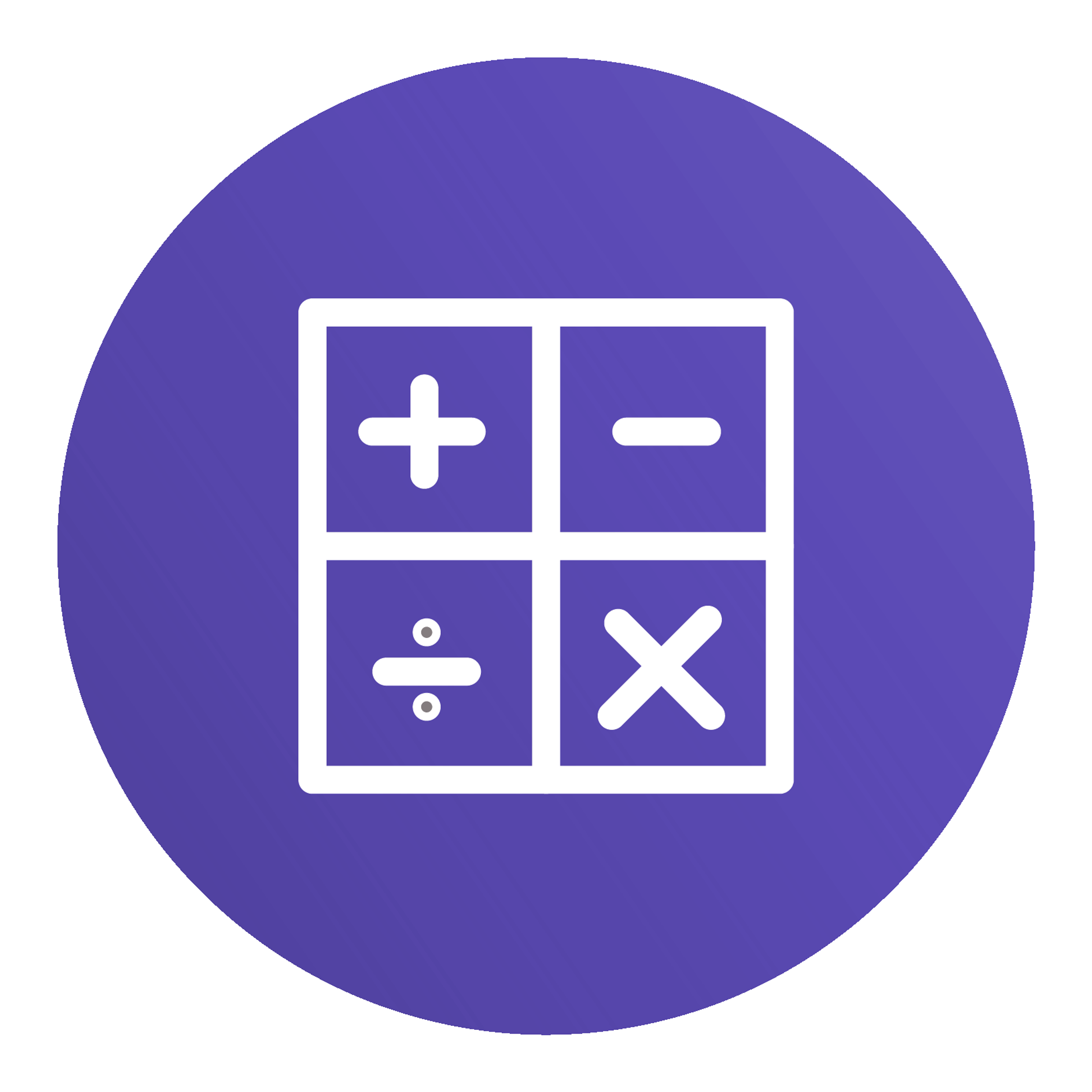# Learning Assistance Math Classes## Philosophy:

To provide students an environment to develop knowledge, skills and tools necessary to succeed in the mathematics found in academics as well as everyday life.

### Classes:

LERN 48- Basic Math Skills Review

3 Units (Not Degree Applicable)
May be taken for Pass/No Pass only

Math fundamentals: adding, subtracting, multiplying and dividing whole numbers and adding, subtracting and multiplying decimals. Emphasis on math learning strategies such as organization and managing math anxiety.

LERN 49- Math Skills Review

3 Units (Not Degree Applicable)
May be taken for Pass/No Pass only
Prerequisite: LERN 48 or or  appropriate placement on assessment measure.

Improves knowledge of basic math. Includes operations and applied problems in whole numbers, fractions, decimals, proportions, percents, and integers. Covers math study strategies such as learning preferences and self-assessment.

### Student Resources for Math:

Math facts (addition, subtraction,multiplication, and division) are fundamental to learning math.  If you have not mastered your multiplication facts you will need to work on accomplishing this.